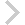FICO Xpress Optimization Examples Repository
 FICO Optimization Community FICO Xpress Optimization HomeOverview of Mosel examples for 'Business Optimization' book

Description

List of FICO Xpress Mosel implementations of examples discussed in the book 'J. Kallrath: Business Optimization Using Mathematical Programming - An Introduction with Case Studies and Solutions in Various Algebraic Modeling Languages' (2nd edition, Springer, Cham, 2021, DOI 10.1007/978-3-030-73237-0).

List of provided model files

(Examples marked with * are newly introduced in the 2nd edition, all other models have been converted from the mp-model versions that were provided with the 1st edition of the book in 1997.)
absval.mos absval2.mos, absval2a.mos bench101.mos bench102.mos Filename Description Section Modeling absolute value terms (linearization) 6.5 * Modeling absolute value as general constraints 6.5 Parkbench production planning problem (solution to Exercise 10.1) 10.9 Parkbench production planning problem, MIP problem (solution to Exercise 10.2) 10.9 Ore blending problem 2.7.1 * Boat renting problem (solution for Exercise 3.3) 1.4.1, 3.7 * Multi-period Boat renting problem 1.6 Dual of the Boat renting problem (solution for Exercise 3.3) 3.7 Brewery production planning (data files: brewery.xlsx, brewdata.dat) 8.3 Generalized assignment problem (solution for Exercise 7.3) 7.10 Small knapsack problem 7.1.1 Bus crew scheduling 7.8.4 Calves and pigs problem 3.3.1 Carton production scheduling problem (data file: carton.dat) 10.3 * Minimal perimeter convex hull for two triangles 10.3 * Contract allocation problem with semi-continuous variables 10.2.1 Feasibility puzzle problem (solution for Exercise 6.5) 6.11 Data envelopment analysis (solution for Exercise 5.2) 5.3 Dual problem for a small LP (solution for Exercise 3.1b) 3.5.1 * Dynamic computation of big-M coefficients for production planning 14.1.2.1 * Production planning problem formulation using indicator constraints 14.1.2.1 * Evolutionary algorithm for supply management 14.1.3.3 Choosing investment projects (solution for Exercise 7.5) 7.10 Flowshop scheduling problem (solution for Exercise 7.7) 7.10 * Fractional programming example 11.1 Generalized assignment problem (solution for Exercise 7.2) 7.10 * Lexicographic Goal Programming 5.4.3 * Lagrange relaxation applied to the GAP 14.1.3.3 Multi-period, multi-site production planning, LP model (solution for Exercise 5.1) 5.3 Multi-period, multi-site production planning, MIP model (solution for Exercise 6.7) 6.11 * Production scheduling problem with SOS formulation (solution for Exercise 6.9) 6.11 Multi-knapsack problem (solution for Exercise 7.4) 7.10 Network flow problem (solution for Exercise 4.3) 4.7 Generic formulation of network flow problem (Exercise 4.3) 4.7 * Newsvendor problem: 2-stage stochastic programming 11.3.2.1 Net present value problem (solution for Exercise 6.8) 6.11 * Optimal breakpoints for piecewise linear approximation 14.2.3 * Multi-stage stochastic portfolio investment model 11.3.2.5 Primal problem for a small LP (solution for Exercise 3.1a) 3.7 Simple production planning example 2.5.2 Project scheduling case study 10.2.3 Linearized quadratic programming example 11.4 * Quadratic programming example solved as NLP 11.4 Set covering problem (solution for Exercise 7.6) 7.10 Simple LP problem (solution for Exercise 2.2) 2.13 Simple LP problem (solution for Exercise 2.3) 2.13 Lifting slabs (solution for Exercise 6.6) 6.11 Sludge production planning example illustrating recursion 11.2.1 * Recursion example solved as NLP 11.2.1 * (solution for Exercise 6.4) 6.11 Trimloss problem LP formulation (solution for Exercise 4.1) 4.1.1 Trimloss problem MIP formulation (solution for Exercise 4.2) 4.1.2 * Trimloss problem formulated as a MINLP problem 13.3 * Alternative NLP solver choice for trimloss problem 13.3 Traveling salesman problem (solution for Exercise 7.1) 7.10 * Vehicle routing - heating oil delivery problem 7.2.3 * (solution for Exercise 2.1) 2.13 Yield management, financial modeling 8.4.2

Source Files

Data Files

dea.mos

(!*********************************************************************
Mosel Example Problems
======================

file dea.mos

DEA model

Example solution to exercise 5.2 in section 5.3 of
J. Kallrath: Business Optimization Using Mathematical Programming -
An Introduction with Case Studies and Solutions in Various Algebraic
Modeling Languages. 2nd edition, Springer Nature, Cham, 2021

author: S. Heipcke, June 2018

(c) Copyright 2020 Fair Isaac Corporation

you may not use this file except in compliance with the License.
You may obtain a copy of the License at

Unless required by applicable law or agreed to in writing, software
WITHOUT WARRANTIES OR CONDITIONS OF ANY KIND, either express or implied.
See the License for the specific language governing permissions and

*********************************************************************!)

model 'dea'
uses "mmxprs"

declarations
UNITS: set of string                 ! Set of locations
OUTPUTS: set of string               ! Set of products
VAL: array(UNITS,OUTPUTS) of real    ! Sales values
COST: array(UNITS) of real           ! Total costs per location
COEF: array(OUTPUTS) of real         ! Sales/total_cost(u)
end-declarations

VAL::(['Paris','London','Bonn'],['A','B','C'])[50,50,50,50,100,40,100,90,60]
COST::(['Paris','London','Bonn'])[110,150,200]

declarations
w: array(OUTPUTS) of mpvar          ! Weights
end-declarations

forall(u in UNITS) do
writeln("****Solving for ", u)
! Calculate coefficients
forall(j in OUTPUTS) COEF(j):=VAL(u,j)/COST(u)

! Objective: Maximise efficiency for unit u
Effcy:=sum(j in OUTPUTS) COEF(j)*w(j)

! Efficiency must not exceed 1
forall(i in UNITS)
Cstr(i):= sum(j in OUTPUTS) VAL(i,j)*w(j)<= COST(i)
! Lower limit on weights
forall(j in OUTPUTS) Lim(j):=w(j)>= 0.001

maximise(Effcy)
writeln("Solution: Efficiency(", u, ")=", getobjval)
forall(i in UNITS-{u}) writeln("  ", i, ":", Cstr(i).act/COST(i))
forall(j in OUTPUTS) writeln("  ", j, ":", w(j).sol)
end-do
end-model